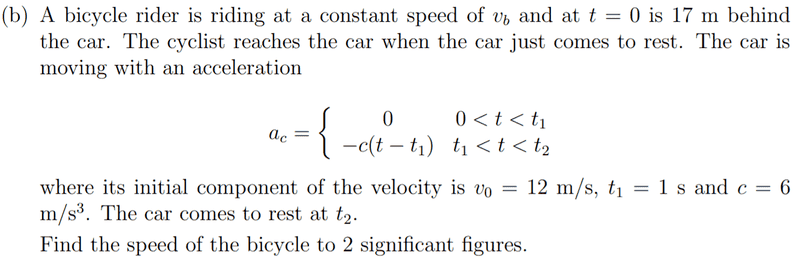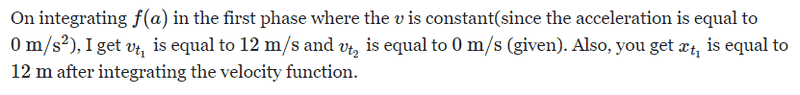# What is the speed of the bicycle?

• brochesspro
In summary, you seem to have pasted in an image containing your work rather than typing in your work directly. This is a common mistake and can make it difficult to understand what you are doing.

#### brochesspro

Homework Statement
Find the speed of the bicycle using the given data to up to 2 significant figures.
Relevant Equations
I am not understanding how to proceed. I could use a hint for calculating the distance travelled by the car when the car is decelerating and then calculating the distance and speed of the bike will be elementary and can be solved easily. Thanks(or sorry for a possible mistake in phrasing or formatting my question) in advance.How far did you get with this? It looks a bit tricky.

PeroK said:
How far did you get with this? It looks a bit tricky.
Use basic integration, specifically the polynomial rule and the fundamental theorem of calculus.

brochesspro said:
Use basic integration, specifically the polynomial rule and the fundamental theorem of calculus.
Where did you get stuck?

You seem to have pasted in an image containing your work rather than having typed in your work directly. Let me transcribe it for you.
brochesspro said:
On integrating f(a) in the first phase where the v is constant (since the acceleration is equal to 0 m/s2, I get ##v_{t_1}## is equal to 12 m/s and ##v_{t_2}## is equal to 0 m/s (given). Also you get ##x_{t_1}## is equal 12 m after integrating the velocity function.
I agree that ##v_{t_1} = v_{t_2} = 12 m/s##.
I agree that ##x_{t_1}## is equal to 12 meters. This is 12 m/s multiplied by 1 s. That integration is trivial.

Given that starting point, what prevents you from writing down a formula for ##v(t)## that is valid for ##t_1 \le t \le t_2##?

Worst case, what prevents you from drawing the velocity/time graph on graph paper and counting squares?

Like I said, I got stuck in the phase of motion during which the car undergoes deceleration. You will be able to understand better if you read the questions.

brochesspro said:
Like I said, I got stuck in the phase of motion during which the car undergoes deceleration. You will be able to understand better if you read the questions.
I have read the question. Where did you get stuck, precisely?

jbriggs444 said:
You seem to have pasted in an image containing your work rather than having typed in your work directly. Let me transcribe it for you.

I agree that ##v_{t_1} = v_{t_2} = 12 m/s##.
I agree that ##x_{t_1}## is equal to 12 meters. This is 12 m/s multiplied by 1 s. That integration is trivial.

Given that starting point, what prevents you from writing down a formula for ##v(t)## that is valid for ##t_1 \le t \le t_2##?
You have not used LaTeX properly so it is hard to understand.

brochesspro said:
You have not used LaTeX properly so it is hard to understand.
It is proper. I fixed one closing ## and it is all perfect.

You should use "$$", not ## Never mind. brochesspro said: You should use "$$", not ##
Embedded ##\LaTeX## works just fine, thank you. With the forum software here, $$starts the LaTeX on a separate line while ## displays in-line. But yes, refresh is sometimes necessary. It was not coming properly before, so I refreshed the page. jbriggs444 said: You seem to have pasted in an image containing your work rather than having typed in your work directly. Let me transcribe it for you. I agree that ##v_{t_1} = v_{t_2} = 12 m/s##. I agree that ##x_{t_1}## is equal to 12 meters. This is 12 m/s multiplied by 1 s. That integration is trivial. Given that starting point, what prevents you from writing down a formula for ##v(t)## that is valid for ##t_1 \le t \le t_2##? Worst case, what prevents you from drawing the velocity/time graph on graph paper and counting squares? I am supposed to use integration. I am unable to use an equation of motion using any three variables. I have ##v_0=12 m/s## and ##v=0 m/s##. brochesspro said: I am supposed to use integration. I am unable to use an equation of motion using any three variables. I have ##v_0=12 m/s## and ##v=0 m/s##. So, what precisely is stopping you? It's not too hard. My suggestion is to simplify things by defining:$$a(T) = -cT \ \ \ (0 < T < T_2) where ##T = t - t_1## That let's you calculate ##T_2## and ##x(T_2)## more easily. Then just add on the initial distance before the deceleration phase.

•jbriggs444
Should it not be ##(T_1<T<T_2)##

brochesspro said:
Should it not be ##(T_1<T<T_2)##
##T_1 = 0##

The first thing you need to do is get an expression for ##v(T)## (or ##v(t)##, if you don't like my approach).

Can you do that?

It's difficult to help if we can't see where you're having the problem.

I am just having a problem in 2
PeroK said:
The first thing you need to do is get an expression for ##v(T)## (or ##v(t)##, if you don't like my approach).

Can you do that?

It's difficult to help if we can't see where you're having the problem.
I am just having a problem in two things, namely:
1. How to find the difference ##t_2-t_1## to find the acceleration using equations of motion, or finding the acceleration directly using the given constant ##c##, and
2. Where and how to use the constant ##c##(for integration) .

I think it will be easy to use the equations of motion rather than use calculus.

brochesspro said:
I think it will be easy to use the equations of motion rather than use calculus.
If you mean SUVAT, they are only valid for constant acceleration. There's no alternative to calculus in this case.

brochesspro said:
find the acceleration using equations of motion
The acceleration is given in the problem statement. You don't need to compute it.

PeroK said:
If you mean SUVAT, they are only valid for constant acceleration. There's no alternative to calculus in this case.
Oh yeah, you are right.

jbriggs444 said:
The acceleration is given in the problem statement. You don't need to compute it.
Yes, you are right. I integrated ##f(a)## for the second phase by putting the values and got a quadratic equation and then I solved it and got ##t_2 = 3s##.

Also, I integrated the speed function (integrated ##f(a)## twice) and got ##x_{t_2} = 10 m##. How is that possible since the car is stopping while going forward and so ##x_{t_2}>12m##.

Could you once check my work on this problem? I am sorry you have to download it.

brochesspro said:
Could you once check my work on this problem? I am sorry you have to download it.

##2.6 m/s##

brochesspro said:
##2.6 m/s##
I thought the bicycle started ##17m## behind the car at ##t = 0##?

##26 m/s## would be a better guess.

PeroK said:
I thought the bicycle started ##17m## behind the car at ##t = 0##?

##26 m/s## would be a better guess.
Be realistic. How can a bicycle go at that speed?

•PeroK
brochesspro said:
Be realistic. How can a bicycle go at that speed?
Perhaps it's Mark Cavendish?

PeroK said:
Perhaps it's Mark Cavendish?
Who is he?

brochesspro said:
Who is he?
Never mind, not even he can ride a bicycle at that speed.